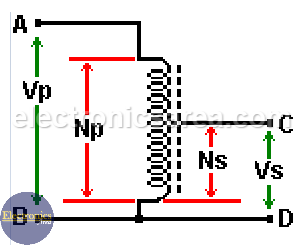# What is an Autotransformer?

An autotransformer or auto transformer is a transformer where a part of the winding belongs to both the primary winding and secondary winding of the transformer. Its operating principle is the same as the conventional transformer, then the relation between input and output voltages, input and output currents and the ratio of number of turns between the primary and the secondary winding is the same.

The currents of the primary winding and secondary winding are flowing on opposite directions, so the total current flowing through the common part of the winding is equal to the difference between the current on the low-voltage winding and the current on the high-voltage winding.

In order for  an auto transformer to work properly, both winding should have the same winding sense. The transformation ratio of this transformer is close to “1”.

## Step-down autotransformer

If an alternating voltage is applied to points A and B, and the output voltage is measured at points C and D, a lower voltage is obtained. This transformer is a step-down auto transformer.  In this case, the turn ratio = Ns/Np < 1.## Step-up autotransformer

If an alternating voltage is applied to points C and D, and the output voltage is measured at points A and B, a higher voltage is obtained. This transformer is a step-up autotransformer. In this case, the turns ratio is: Ns/Np > 1.• Autotransformers have a lower weight and a lower cost.
• Instead of having a high voltage winding of N1 turns, it must be provided, for low voltage winding, with a number N2 of turns. An additional number of turns of N1 – N2.
• It must also be taken into account that the wire of the common winding section must have a copper cross-section that depends on the current difference between low voltage winding and high voltage winding.
• Another advantage is that there is no separation (isolation) between the primary winding and secondary winding. However, this brings the disadvantage that the primary winding is not independent of the secondary one.
• This kind transformer can be dangerous for a person, because there is the primary winding voltage between the ground connection and the common wire part of the secondary winding and primary winding. See the step-down auto transformer diagram.

• The load could be directly connected to the high voltage source if the insulation between both winding of this transformer fails.
• It has a high short circuit current.

## Applications of an autotransformer

• Soft start (reduced voltage) for squirrel cage motors, to avoid high current demand, at startup. Thus at startup it is applied from 50% to 60% of the primary voltage.
• It allows compensating the voltage drop in distribution cables in electrical installations over long distances (eg in rural areas)
• To interconnect transformers in 132kV / 330kV systems, for example.

## Conventional transformer connected as an auto transformer

The connection is shown in the following images, where:

• To obtain a “step-down autotransformer” using a conventional transformer, the lower terminal of the primary winding must be connected to the upper terminal of the secondary winding and from this connection point the output of the transformer is obtained.
• To obtain a “step-up autotransformer”, the lower terminal of the primary winding must be connected to the upper terminal of the secondary winding. The input in this case is at the junction of both winding and the output is at the upper terminal of the primary winding.

Take into account that two other possible variations of this transformer can be obtained if Np and Ns are exchanged in each of the previous diagrams.

With this type of connections, the ability to transmit power is improved and there is also a better power factor.

• 29
•
•
•
•
•
•
•
•
29
Shares
•
29
Shares
• 29
•
•
•
•
•
•
•

### One Response to “Autotransformer”

1.Super